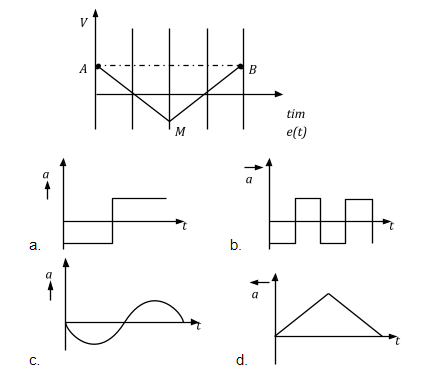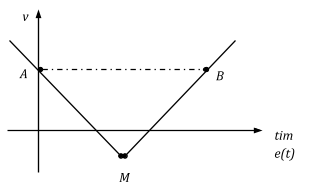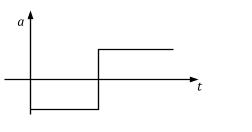# If the velocity-time graph has the shape AMB, what would be the shape of the corresponding acceleration-time graph?a= dv / d t= slope of (v – t) curve

If m = +ve, then equation of straight line is

y = mx + c ⇒ v = mt + c (for MB)

If m = –ve, then equation of straight line is

y = –mx + c ⇒ v = -mt + c (for AM)

If we differentiate equation (1) and (2), we get

asub>MB = +ve = m where m is constant.

asub>AM = –ve = –m, so graph of (a-t) will be(1)(0)